# What Is Trigonometry? Definition, Formulas & Applications

Trigonometry is a branch of mathematics that deals with the study of triangles. It is sometimes informally referred to as "trig." In trigonometry, mathematicians study the relationships between the sides and angles of triangles. Right triangles, which are triangles with one angle of 90 degrees, are a key focus of study in this area of mathematics.

Trigonometry is widely taught in high schools, typically to students in their junior year. Trigonometry builds on basic math concepts learned in lower grade levels, and it can be a confusing subject to navigate. Trigonometry tutors can either help students get a better grasp on trigonometry concepts or challenge them to think outside of the box, depending on their academic level and understanding of trigonometry. Read on to find out more about the invention of trigonometry, the best way to master it, how tutors can help, and how trig is different from other math subjects.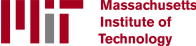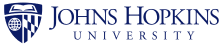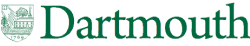## What Does Trigonometry Mean?

The word trigonometry originates from the Greek words trigonon and metron, which mean "triangle" and "measure," respectively. The basics of trigonometry may be even older, and may have been used in ancient Egypt. Essentially, trigonometry deals with triangles and how the sides and angle measurements of a triangle relate to one another.

There is a wide range of careers that require the use of trigonometry, including architect, astronaut, crime scene investigator, physicist, surveyor, engineer, and many more. Even if you aren’t planning to pursue a career in any of these fields, it's still important to understand basic trigonometry concepts. Trigonometry is a required class in most school districts, and if students don't do well in this subject, it can reflect poorly on their overall academic record. There are several reasons why trigonometry is currently taught in schools, and many ways in which trigonometry can be used and applied in later life.

## Where Does Trigonometry Come From?

Trigonometry has a long history, dating back to the ancient world. Initially, trigonometry was concerned with utilizing basic functions to use the known angle of a triangle in order to calculate the remaining angles. For example, if two side lengths of a triangle were given and the measure of the enclosed angle was known, the third side and the two other angles could be calculated. Trigonometry, both then and now, is primarily focused on the relationships between the angles of triangles.

The ancient Greeks formalized the first trigonometric functions, starting with Hipparchus of Bithynia, circa 150 B.C. Hipparchus considered every type of triangle, including spherical triangles, right-angled triangles, and planar triangles. While Hipparchus's interest in trigonometric functions was  mainly related to astronomy, trigonometry in modern times can be applied to many real-world situations.

## What Is Trigonometry Used For?

As previously mentioned, trigonometry is often utilized in fields related to physics, chemistry, and engineering. Far from simply being a theoretical subject, there are numerous practical applications of trigonometry. Engineers in many industrial fields use trig in the course of their work. Other professionals that may use trigonometry include surveyors, architects, and pilots. Just as Hipparchus did over two thousand years ago, trigonometry is used in astronomy to measure the distance of nearby stars and to calculate stellar positions. Trigonometry also plays a key role in satellite navigation systems.

One real-life problem that can be solved using the rules of trigonometry is to work out the measurements of things or spaces that would be difficult to measure directly. For example, trigonometric functions can be used to calculate the heights of mountains, the quantity of water in a lake, and the square footage of an unusually-shaped piece of land. Trigonometry can even be used to help astronomers measure time accurately.

Trigonometry concepts are used in gaming and music, as well. Trigonometry plays a role in video game development by ensuring that games function properly. It’s used to  write programs so that objects in the game can move. Trigonometry can also be utilized in the process of designing characters, sets, and objects for video games. In music, trigonometry measures the level or pitch of a sound wave or musical note.

Thus, although trigonometry is certainly a math concept, it can be applied to many different fields and tasks outside of mathematics. These are all real-life scenarios in which a person would use trigonometry, and they’re examples of why it’s important to learn how to apply trigonometry correctly.

## When Do You Learn Trigonometry?

Because trigonometry can be applied to careers in many different fields, it’s important to ensure that students have a good grasp of trig concepts. Trigonometry builds on basic math knowledge taught throughout a student’s education, including algebra and geometry concepts. Because trigonometry combines concepts from different math subjects, there is a specific order in which these subjects are taught.

Typically, students take Algebra I, Geometry, and then Algebra II or Trigonometry. Depending on the state, some schools offer students a choice of which math subject they’d like to study. A student’s ability to choose a specific math subject may also depend on their academic performance. Since trigonometry applies concepts taught in earlier grade levels and builds upon previous knowledge, it can be a tough subject to tackle.

## Can You Learn Trigonometry Online?

With the rise of online learning, there have been amazing advances in how virtual classrooms function. There are many ways that online education can help all learners to master trigonometry. By using educational apps and software, teachers can share their computer screens and show exactly how to solve math problems, just as they would use a whiteboard or chalkboard to work out problems for students in the classroom.

Online classes are often smaller than in-person classes, allowing teachers to focus on assisting students who need help with specific trigonometry concepts. Online education has developed significantly in the last few years and now offers a safe space for all types of learners and learning styles.

## Is Trigonometry Hard?

While math comes naturally to some, trigonometry can be a confusing topic for many people. When you consider that a trigonometry class may involve trigonometric functions, trigonometric values, trigonometric formulas, and much more, it can be  overwhelming and confusing to keep all of these components straight. The best way to ensure that students are using tangent, sine, and cosine functions correctly is to have guidance from someone with a detailed understanding of trigonometry. This could be a classmate, parent, teacher, or tutor.

﻿Even if a student can perform algebraic functions and has a good grasp of concepts in other branches of mathematics, they might struggle with certain elements of trigonometry, such as the trigonometric ratios sine (sin), cosine (cos), and tangent (tan). Regardless of whether a student learns in a face-to-face classroom or a virtual classroom, it can be very helpful to have one-on-one help from a tutor. That way, students can receive immediate feedback. A trigonometry tutor can help students feel confident about the new concepts they’re encountering and can reinforce what they’re learning by providing extra practice.

## How Learner Trigonometry Tutors Can Help

Trigonometry can be a daunting subject, especially for people who don’t naturally excel in math or science. For parents, it can be tough to expect their children to excel in every subject. It’s worth remembering that there are many different types of learners and many different learning styles. Some people learn best with visuals, some people learn best by hearing the information, and some people learn best when they can read and listen to the instructions for a specific task.  Some students may benefit from the help of an online math tutor. Tutors can also help students who are performing well academically by offering extra practice before college prep tests or school exams.

Many education-related businesses claim to help students, but it can be difficult to distinguish between companies that see young people as dollar signs and those that genuinely want students to succeed. It can also be challenging to find a tutor who fits a family’s busy schedule. Luckily, there are platforms that offer excellent online tutors who are custom-matched to students through a rigorous screening process. One of the best known is Learner. Learner focuses on providing specific academic instruction that is tailored to each student, rather than supplying generic lessons to all students.

Learner is so confident that they can meet their customers’ tutoring needs that they have a 100% risk-free satisfaction guarantee. Learner’s innovative platform offers collaborative tools to boost engagement, feedback metrics that let students know where they stand, and session recordings so that students can review later. and Learner works across all devices. Learner is one of many online tutoring websites, but it’s one of the only platforms that uses personalized matching with world-class tutors to drive accelerated learning.

Need help with trigonometry? Speak with our academic advisor to get matched with a top online math tutor today!

### Is Trigonometry Geometry?

Geometry is the branch of mathematics that studies the properties and relations of points, lines, surfaces, and solids, whereas trigonometry focuses exclusively on angles and triangles. Geometry is often taught in schools before trigonometry.

### Is Trigonometry Precalculus?

Trigonometry is a prerequisite to precalculus courses because precalculus uses elements of trigonometry, algebra, and analytical geometry.

### Is Algebra 2 Trigonometry?

Algebra 2 does not teach the same math concepts as trigonometry. Algebra 2 covers topics such as linear equations, functions, quadratic equations, polynomials, radical expressions, inequalities, graphs, and matrices. Trigonometry, on the other hand, is concerned with the specific functions of angles and how they are applied to calculations.

### What Are Trigonometric Identities?

Trigonometric identities are equalities involving trigonometric functions. They are important elements in the study of triangles. Trigonometric identities include Pythagorean identities, reduction formulas, and cofunction identities. Often, a scientific calculator is used to solve trig problems such as trigonometric identities.

### What Are Trigonometric Functions?

Trigonometric functions describe the relationships between the angles and sides of a triangle. In modern mathematics, there are six main trigonometric functions, also called trigonometric formulas: sine, tangent, secant, cosine, cotangent, and cosecant. These functions describe the ratios of the sides of right triangles.

### What Is Sin in Trigonometry?

Sine (sin), cosine (cos), and tangent (tan) are the three basic functions used in trigonometry. They’re based on right-angled triangles. The sine of one angle of a right triangle is the ratio of the length of the side of the triangle opposite the angle to the length of the triangle’s hypotenuse.

### What Is Cos in Trigonometry?

Sine (sin), cosine (cos), and tangent (tan) are trigonometric functions based on right-angled triangles. The cosine of an angle in a right triangle is the ratio of the length of the side adjacent to the angle to the length of the hypotenuse.

### What Is the Tangent Function in Trigonometry?

In a right triangle, the tangent of an angle is the ratio of the opposite side to the adjacent side of that angle.

### What Is the Pythagorean Theorem?

The Pythagorean theorem states that for a right-angled triangle, the square of the length of the hypotenuse is equal to the sum of the squares of the lengths of the other two sides.

### Does Trigonometry Work with Non-Right Angles?

Typically, high school trigonometry courses focus on applying trigonometric functions such as sine, cosine, and tangent to right triangles (which have one right angle). More advanced college trigonometry could include these functions as well as the application of trigonometry to non-right angles.

### What Do Trigonometry Courses Cover?

Trigonometry courses typically cover topics such as the Pythagorean identity and how to use trigonometric functions such as sine and cosine to solve right triangles. More advanced courses may include the study of complex numbers, polar coordinates, De Moivre's Theorem, and Euler's Formula.

### What Comes After Trigonometry?

In most schools, the math curriculum follows a specific order. Typically, students take Algebra I, Geometry, Algebra II or Trigonometry, Precalculus, and Calculus. Some students may take honors courses, which means they will begin Algebra I in eighth grade, instead of ninth grade. This would allow them to take Calculus during their senior year, instead of Precalculus. The curriculum in private schools may differ from this order.

## Latest Articles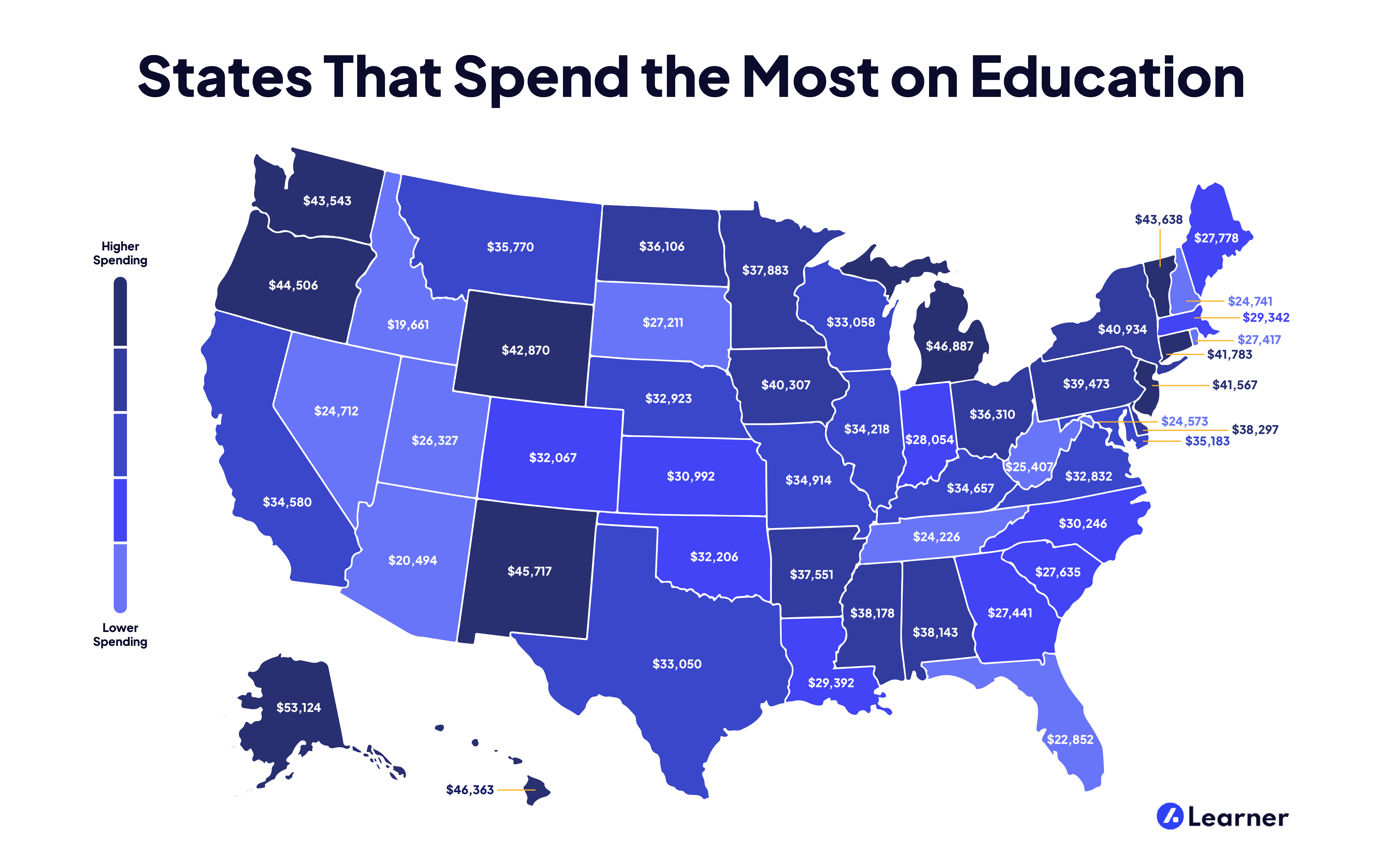### States That Spend the Most on Education

Investing in the future of our children via their education can help them build the lives they want. But education comes at a price—and a high one, at that. And some states invest more in their students than others. Find out which states have the highest spending per student!

Mathematics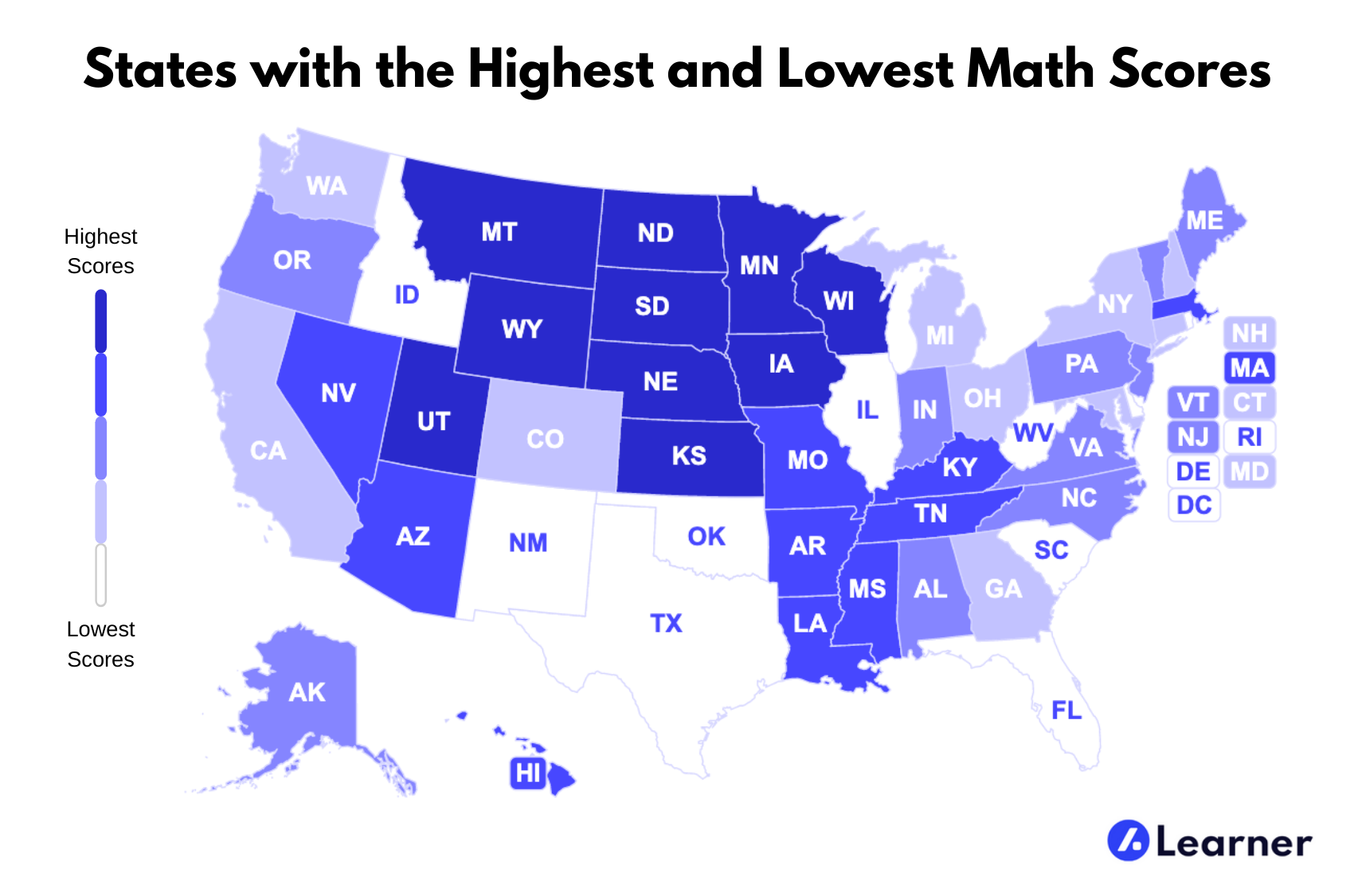### States with the Highest and Lowest Math Scores

At Learner, we understand both the difficulty and the necessity of math as a subject and wanted to know what math looks like in the United States. We took a look at the average SAT math scores throughout the nation to find which states had the highest and the lowest. Find out how your state stacks up against the rest!

Mathematics### How Can I Score Well on the GED® Math Test?

Passing the General Education Development® (GED®) test gives a person the equivalent of a high school diploma. The math test is one of five sections of the exam. To score well on the GED® math test, you need a sound understanding of the concepts tested in each of the four content areas covered in this section.

Mathematics